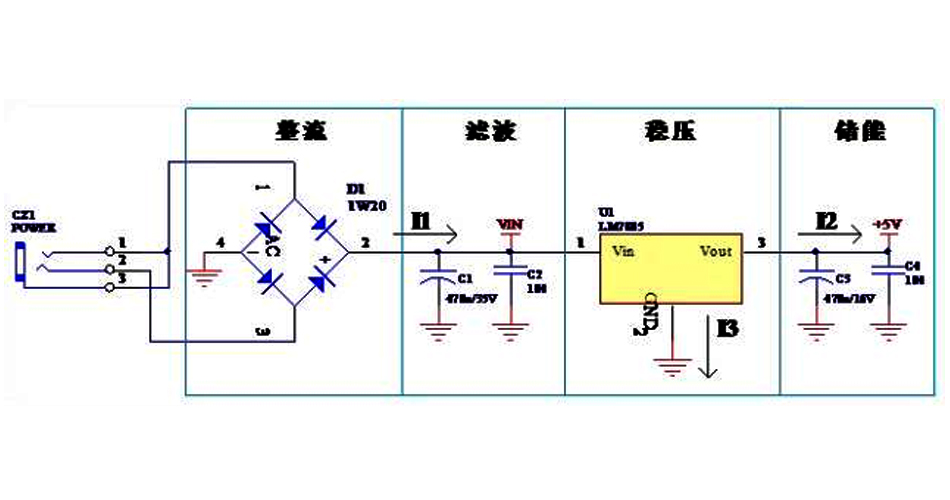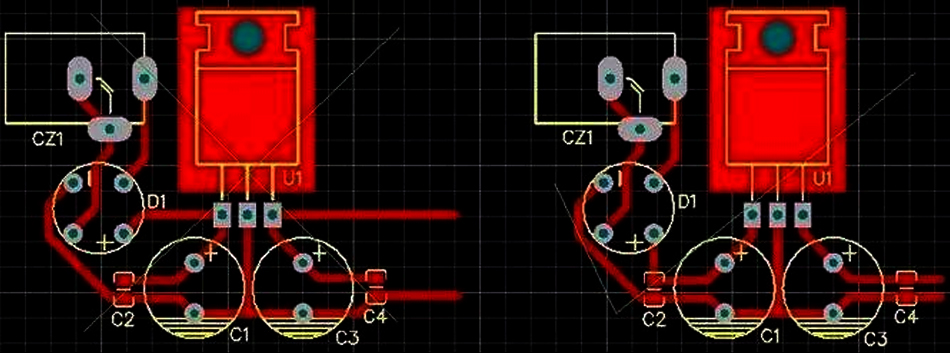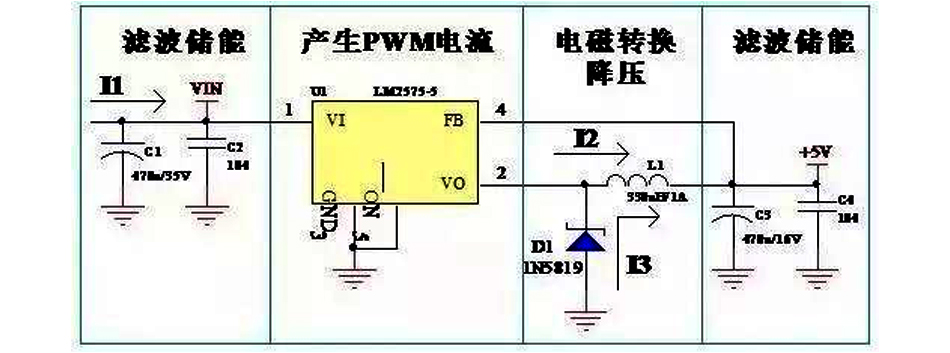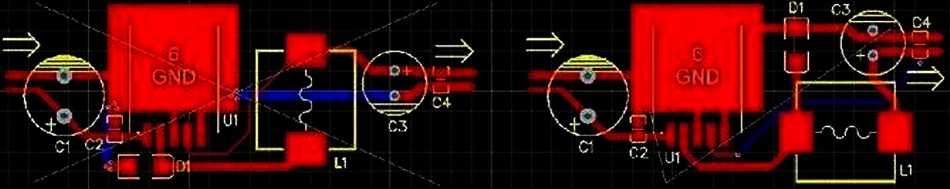# Why PCB Design Should Learn The Design of Power Supply Circuits?

###### Why learn the design of power supply circuits?

Power supply circuit is an important part of an electronic product, the power supply circuit design is good or bad, directly implicated in the performance of the product.

###### Classification of power supply circuits

The power supply circuits of our electronic products mainly include linear power supply and high-frequency switching power supply. Theoretically, the linear power supply is the user needs how much current, the input side to provide how much current; switching power supply is the user needs how much power, the input side to provide how much power.

###### Linear power supply circuit schematic example

Linear power supply power devices work in a linear state, such as our commonly used voltage regulator chip LM7805, LM317, SPX1117, etc.. Figure 1 below shows the LM7805 regulated power supply circuit schematic.Figure 1 Linear power supply schematic diagram

As can be seen from the figure, the linear power supply has a rectifier, filter, voltage regulator, energy storage and other functional components, at the same time, the general use of the linear power supply for the series regulated power supply, the output current is equal to the input current, I1 = I2 + I3, I3 is the reference end, the current is very small, so I1 ≈ I3. why do we need to talk about the current, because when the PCB is designed, the width of each line is not randomly set up, it is based on the schematic diagram in the component nodes to determine the current size. Current size, current flow direction to be clear, do the board just right.

###### Linear power supply PCB diagram

PCB design, the layout of the components should be compact, to make all the lines as short as possible, according to the schematic component function relationship to layout components and alignment. This power supply diagram is the first rectifier, then filtering, filtering before the voltage regulator, voltage regulator before the energy storage capacitor, flow through the capacitor before the back of the circuit with electricity.

Figure 2 is the PCB diagram of the above schematic, the two diagrams are similar. Left and right is a little different alignment, the left figure of the power supply rectified directly to the input pin of the voltage regulator chip, and then the regulator capacitor, where the capacitor plays a filtering effect is much worse, the output is also a problem. The picture on the right is a better one. We not only have to consider the flow of the positive power supply, but also have to consider the ground return problem, in general, the positive power line and ground return line as far as possible with the same in and out, close to each other.Figure 2 linear power supply PCB diagram

Design of linear power supply PCB should also pay attention to the linear power supply power regulator chip heat dissipation problems, heat is how to come, if the regulator chip front-end voltage is 10V, the output side is 5V, the output current is 500mA, then the regulator chip on the voltage drop of 5V, resulting in heat for 2.5W; if the input voltage is 15V, the voltage drop is 10V, resulting in heat for 5W! Therefore, we cloth board is to be based on the thermal power to leave enough cooling space or a reasonable heat sink. Linear power supply is generally used in the pressure difference is relatively small, the current is relatively small occasions, otherwise, please switch to switching power supply circuit.

###### High frequency switching power supply circuit schematic example

Switching power supply is used to control the switching tube through the circuit for high-speed conduction and cut-off, generating PWM waveforms, through the inductor and the continuity diode, the use of electromagnetic electric conversion of voltage regulation. Switching power supply power, high efficiency, low heat, we generally use the circuit: LM2575, MC34063, SP6659 and so on. Switching power supply theory is equal power at both ends of the circuit, the voltage is inversely proportional to the current is inversely proportional.Figure 3 LM2575 switching power supply circuit schematic diagram

###### Switching power supply PCB diagram

Switching power supply PCB design, need to pay attention to the place is: the introduction point of the feedback line, the renewal diode is to whom the renewal. As can be seen from Figure 3, U1 conduction, the current I2 into the inductor L1, the characteristics of the inductor is that the current flowing in the inductor can not be suddenly generated, and can not be suddenly disappeared, the current changes in the inductor when there is a time course. In the role of pulse current I2 flow through the inductor, part of the electrical energy into magnetic energy, the current gradually increased to a certain time, the control circuit U1 shut down I2, due to the characteristics of the inductor, the current can not suddenly disappear, this time the diode plays a role in the diode, which takes over the current I2, so it is called a diode to continue the diode, you can see that the diode to continue the diode to the inductor, continue the current I3 is from the negative end of the C3 departure , through D1, L1 flows into the positive end of C3, here is equivalent to pumping, the use of inductive energy, the capacitor C3 voltage. There is also the introduction of voltage detection of the feedback line point problem, should be filtered after the place of feedback back, otherwise it will make the output voltage ripple greater. These two points are often ignored by many of our PCB designers, thought the same network, connected there is not the same, in fact, not the same place, the performance impact is very large. Figure 4 is the LM2575 switching power supply PCB diagram, we look at the wrong one is where the wrong.Figure 4 LM2575 switching power supply PCB diagram

Why do we need to talk in detail about the principle of the schematic, because the schematic contains a lot of information to draw the PCB, such as component pin access points, node network current size, etc., to see the schematic clearly, PCB design will not be a problem. LM7805 and LM2575 circuits represent a linear power supply and switching power supply typical board circuit, do PCB, directly according to the layout of these two kinds of PCB diagrams And wiring on the line, just different products, circuit boards are different, according to the actual situation.

Scroll to Top